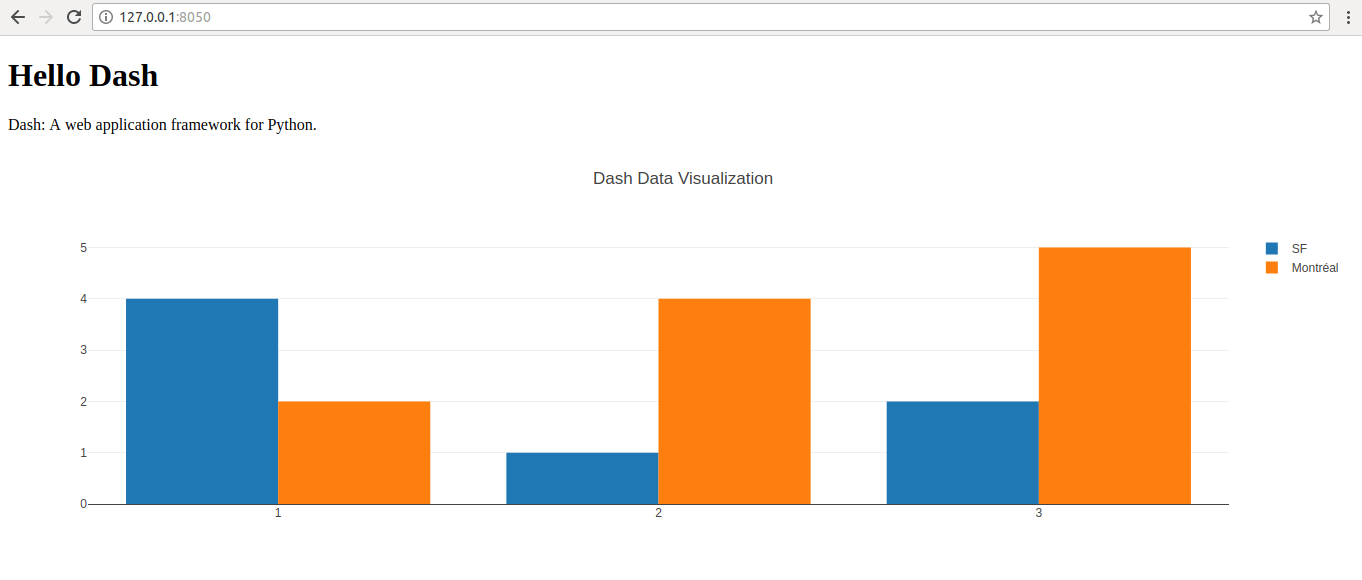# Simple graphs using Dash

In previous post, getting started with Dash we saw how to get simple Dash site up and running. We set up Dash site without any graph, now let us add simple graphs to the side.

Simple Bar Chart with Dash

Following is the code for simple Dash Bar chart site.


# -*- coding: utf-8 -*-
import dash
import dash_core_components as dcc
import dash_html_components as html

app = dash.Dash()

app.layout = html.Div(children=[
html.H1(children='Hello Dash'),

html.Div(children='''
Dash: A web application framework for Python.
'''),

dcc.Graph(
id='example-graph',
figure={
'data': [
{'x': [1, 2, 3], 'y': [4, 1, 2], 'type': 'bar', 'name': 'SF'},
{'x': [1, 2, 3], 'y': [2, 4, 5], 'type': 'bar', 'name': u'Montréal'},
],
'layout': {
'title': 'Dash Data Visualization'
}
}
)
])

if __name__ == '__main__':
app.run_server(debug=True)


Here is the outputIf you compare this code with the previous post code, you will see only one different. We have added “dcc.Graph” component here.

This is where magic happens. You can add multiple dcc.Graph cmponents to display multiple graphs on the same page.

Let us look at simple line graph. Please note the only different between bar chart and line chart.


# -*- coding: utf-8 -*-
import dash
import dash_core_components as dcc
import dash_html_components as html

app = dash.Dash()

app.layout = html.Div(children=[
html.H1(children='Hello Dash'),

html.Div(children='''
Dash: A web application framework for Python.
'''),

dcc.Graph(
id='example-graph',
figure={
'data': [
{'x': [1, 2, 3], 'y': [4, 1, 2], 'type': 'bar', 'name': 'SF'},
{'x': [1, 2, 3], 'y': [2, 4, 5], 'type': 'bar', 'name': u'Montréal'},
],
'layout': {
'title': 'Dash Data Visualization'
}
}
)
])

if __name__ == '__main__':
app.run_server(debug=True)


Here is the outputNow let us look at scatter plot


import dash
import dash_core_components as dcc
import dash_html_components as html
import pandas as pd
import plotly.graph_objs as go

app = dash.Dash()

import numpy as np

N = 1000
random_x = np.random.randn(N)
random_y = np.random.randn(N)
random_x2 = np.random.randn(N)
random_y2 = np.random.randn(N)

app.layout = html.Div([
dcc.Graph(
id='life-exp-vs-gdp',
figure={
'data': [
go.Scatter(
x = random_x,
y = random_y,
mode = 'markers',

)
],
}
)
])

if __name__ == '__main__':
app.run_server()


Output is as belowThis site uses Akismet to reduce spam. Learn how your comment data is processed.